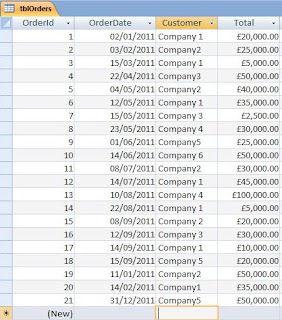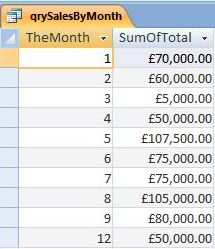## Friday, 16 September 2011

### Manipulating Dates Using the DatePart Function

I was recently asked a question on my Access 2007 Tutorial Facebook Page which involved the manipulation of dates.  In my answer to this question, I suggested a solution that made use of the DatePart Function.  If you are interested, I was replying to NorAzri's comment on his post of the 5th Sep 2011.  However, manipulating dates in this way is quite an interesting area, so I thought I would use this blog to write a bit more about the function.

So what does DatePart actually do?  Put simply, the DatePart Function allows us to isolate part of a given date.  In so doing we may then go on to group or retrieve records according to the date interval set . We use the function by passing an interval parameter telling Access which part of the date we are interested in, and a second parameter which is the date itself.  The syntax for the function is constructed as follows:

DatePart("Interval", DateValue)

The interval parameter is comprised of the same values as that used in the related DateDiff Function - a function I blogged about in August.  These intervals may pertain to the Year ("yyyy"), the Quarter ("q"), or the month ("m") to name but three. There are ten possible interval types in total (going right down to hours, minutes, and seconds).

However, NorAzri was interested in filtering his records by month.  So in order to do this we passed "m" for the interval parameter, and fldDate (a field value from the table) for the date parameter.  The syntax for this was written as follows:

DatePart("m", [fldDate])

The result of the function gave us a numeric month value based on the full date.  For example, if the date in fldDate had been 16/09/2011, the function would have returned the value 9. This is useful when we have a list of dates, such as that in Figure 1 below, which we want to group by month value in a query or report.Figure 1:  List of Orders with dates.

So lets take a look at how we would use the DatePart Function in a query to group these order records by month:Figure 2

As you can see in Figure 2 above, we have created a calculated field called TheMonth using the DatePart Function.  The interval, "m", has been passed in the first parameter, and the second parameter references the OrderDate field from tblOrders.  We have also clicked the TOTALS icon from the SHOW/HIDE group of the DESIGN ribbon, setting TheMonth and Total fields to GROUP BY and SUM respectively.

When we run the Query, any month containing an order will be represented in the results as its own individual row.  The first column then displays the Month value derived from the DatePart function in TheMonth, and the second contains the sum of order totals for the particular month in question.  Figure 3 below shows the query result for our sample data:Figure 3: Results of Query with Calculated Field using the DatePart Function.  SumOfTotal gives us the total for each group of order records falling within each Month.

Another excellent use of DatePart would be to filter a group of records using a criteria based on this function.  For example, if we wanted to show each individual record for all orders falling within the month of May, we would construct our Query as follows:Figure 4
As you can see, we have kept our calculated field called TheMonth.  However, instead of grouping all the records from tblOrders by month, we have added the month value =5 as a criteria for this column.  When we run the query, all Orders from May will be displayed.Figure 5: The result of the second Query filters out all orders from May.

If we wanted, we could convert this to a parameter query, and use it as the data source for a report.  This was something I recommended to NorAzri who wanted to select the Month from a Combo Box on an unbound form, and then produce a report which would filter out records falling within the month in question.  If you are interested in seeing the solution I suggested, just follow the link at the top of this post and look down the stream.